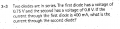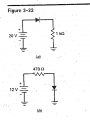# diode circuit (verify answer & help)

#### SLopez

Joined Sep 8, 2014
1
Hey guys so I'm taking an Introductory circuit analysis class for my new major and the professor assigned us hw but he didn't really go that much in depth with circuits so I am not that sure if I did this problem correct if anyone can verify it would be very helpful. Thanksfor 3-3 I did this : first I found the resistance of the resistor since I know that a diode has to always be with a resistor. so R=V/I = .75v/400ma => R=1.875 Now Idiode2= .8v/1.875=.42AmpsFor figure 3-22 a and b we have to find the load current and the load voltage: for a I did this:
Idiode=v/r => 19.3/1k=19.3ma
and the load voltage I think it should be 0v

but for circuit b im not sure if it is the same process can someone explain part b to me.

#### MrChips

Joined Oct 2, 2009
29,861
Your assumptions are incorrect because the I-V characteristic of a diode is highly non-linear and hence does not obey Ohm's Law.

Secondly, the current in circuit (a) cannot be 400mA with the information provided.

#### wmodavis

Joined Oct 23, 2010
739
If the diodes are in series the current through each diode has to be the same i.e. 400mA.

#### MrChips

Joined Oct 2, 2009
29,861
oops... sorry, I see these are separate questions that are not related.

#### wmodavis

Joined Oct 23, 2010
739
I was just taking 3-3 literally for what it says. "Two diodes are in series."
In a series circuit the current flowing through one element must flow through all components in that series circuit.
The voltage drops are irrelevant to the question asked.

•b1u3sf4n09

#### b1u3sf4n09

Joined May 23, 2014
113
For figure 3-22 (a), you found the correct diode current (and as discussed earlier, the circuit current). I believe the load voltage is the voltage drop across the 1 k ohm resistor (the question may illustrate differently). Since you already know the voltage across the diode (0.7 V), it is quite easy to determine the resistor voltage (Hint: you have already determined it in your calculation). Just remember that the resistor now has to drop the remaining voltage potential, post diode, as it is connected to ground (i.e. all remaining voltage must go to 0).

For figure 3-22 (b), you do have to go through the same process, and in fact, you will get a very similar answer. Remember that the diode will drop 0.7V as long as it is in conduction (as long as the anode is 0.7V more positive than the cathode), and we know that the voltage is 0 at ground. Therefore, we know that there is 12V on the left side of the resistor, and there is 0.7V to the right. With that information, you should be able to determine the load current and load potential, or voltage.

Last edited:

#### vk6zgo

Joined Jul 21, 2012
677
wmodavis said:"I was just taking 3-3 literally for what it says. "Two diodes are in series."
In a series circuit the current flowing through one element must flow through all components in that series circuit.
The voltage drops are irrelevant to the question asked"

Exactly!-----what is it about the word "series" that the other posters don't understand?•JoeJester

#### JoeJester

Joined Apr 26, 2005
4,390
The voltage drops are irrelevant to the question asked
The "stem" of the problem was the current in a series circuit. The superfluous information about the voltage drop across the diodes can and will lead people astray. They didn't say two identical diodes.

#### vk6zgo

Joined Jul 21, 2012
677
The "stem" of the problem was the current in a series circuit. The superfluous information about the voltage drop across the diodes can and will lead people astray. They didn't say two identical diodes.
Yeah,Joe,my comment was a bit harsh,as the attachment is misleading.

I wonder if the first question was supposed to be a "gimme"& was just to introduce the concept of diode voltage drops,to see if the student could "run with that"& use the idea in the next question.
If that was the plan,it was poorly implemented.

#### JoeJester

Joined Apr 26, 2005
4,390
I didn't think your comments were harsh, they were spot on. I could have simply "liked" your post or agreed with it, but I chose to talk about stems and superfluous items in the question.

Maybe the first question was to test "comprehension". It's like those questions where the instructions state to "read the instructions carefully before answering any questions" that state "put your name at the top and turn the paper in."